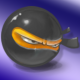Yes, this is how to do 3D lettering (one way, anyway).

It is really hard to do 3D lettering, because setting up vertices for curved letters is very difficult.

But my code can do it for any font of any size.

Of course, I cheated.

That image is not 3D.

Nor is it a single image.

It is 25 images, one behind the other, separated by 0.4 of a pixel. The front image has a brighter colour than the others, to make them look shaded. This creates the illusion of a solid 3D image.

There is one problem I’ll explain below.

You can almost see what I am doing if you look at my setup and draw functions. I put all the detailed code in separate functions (I’m trying the Rapid approach of breaking your code up into little functions that each do one thing).

Underneath, I’ll explain what each function does.

```function setup()
ThreeD=Create3DImage(180)
Settings()
end

function draw()
background(255)
SetupCamera()
ChangeDistance()
pushMatrix()
TranslateRotate()
ReverseRotationIfSideOn()
DrawLayers()
popMatrix()
ChangeRotation()
end
```

### Create3DImage

I create a square flat mesh in memory with “3D” written on it in white. Doing this just makes the drawing faster. (Why white? Because then, I can use the setColors function to alter it to any other colour. If I made it any other colour, then setColors would mix this colour with the new colour, and I don’t want that).

```function Create3DImage(size)
local m=mesh()
local img=image(size,size)
setContext(img)
fill(255)
fontSize(144)
text("3D",size/2,size/2)
setContext()
m.texture=img
return m
end
```

### Settings

I think these are fairly obvious, except nextRotChange. I leave the image facing forward for 5 seconds at the beginning, then I change the rotation randomly (using ChangeRotation) every 15 seconds after that. So nextRotChange just tells me when to do that. You can ignore it and do your own rotations.

```function Settings()
--starting position of image
pos=vec3(0,0,-500)
--starting rotation in x,y,z axes
rot=vec3(0,0,0)
--change in rotation at each frame
dRot=vec3(0,0,0)
--time until we randomly change the rotation
nextRotChange=5
--change in z value at each frame
dZ=1
--number of layers of text and separation between them
LayerCount=25
LayerThickness=0.4
end
```

### SetupCamera

Absolutely standard 3D. The camera is at zero, facing forward into negative z.

```function SetupCamera()
perspective()
camera(0,0,0,0,0,-500)
end
```

### ChangeDistance

At each frame, we move the image closer or further away, reversing direction when we get too close or too far.

```function ChangeDistance()
pos.z=pos.z+dZ
if pos.z<-500 or pos.z>-210 then dZ=-dZ end
end
```

### TranslateRotate

We translate to the current position, and rotate in x,y and z axes (using the rotate function, you have to do them all one by one).

```function TranslateRotate()
translate(pos.x,pos.y,pos.z)
rotate(rot.x,1,0,0)
rotate(rot.y,0,1,0)
rotate(rot.z,0,0,1)
end
```

### ReverseRotationIfSideOn

Here is the one problem of faking 3D with a set of 2D images. If you view them sideon, you see the gaps between the separate images. So this code prevents the rotation from ever showing a sideon view, by reversing the rotation if it gets too close.

```function ReverseRotationIfSideOn()
local v1,v2= modelMatrix()*vec3(0,0,-1),modelMatrix()*vec3(0,0,1)
if math.abs(v1.z-v2.z)<0.1 then dRot=-dRot end
end
```

So I need some way of detecting whether we are close to a sideon view. This is hard, because we are rotating in all three axes, x, y and z, and because you can’t simply add all the rotations so far to see where you are (that gives the wrong answer). Hmm…

But I know that modelMatrix can help me (don’t know what it is? – see here. ).

If I have two pixels, one in the front image and one in the back, then as we get close to a sideon position, the z (depth) value of those pixels will get very close, and it will be exactly the same when we are sideon. So all we need to do is test the z difference between two pixels that start off being one behind the other. I can use the vectors (0,0,-1) and (0,0,1) because we’re only worried about z.

I multiply each of them by modelMatrix(), which tells me what their position will be if we apply the current translation and rotations. Then I compare them, and if they are within 0.1 pixels of each other, I reverse direction.

### DrawLayers

Drawing the layers is pretty simple.

```function DrawLayers()
--draw inside layers
ThreeD:setColors(173, 42, 42)
for i=1,LayerCount do
translate(0,0,LayerThickness)
ThreeD:draw()
end
--draw front layer
translate(0,0,LayerThickness)
ThreeD:setColors(255,0,0)
ThreeD:draw()
end
```

### ChangeRotation

This function changes the rotation randomly. The only reason I put this in was that if the original (random) rotation turned out to be strange, at least it would change. You can leave this out.

```function ChangeRotation()
rot=rot+dRot
--change rotation randomly every 15 seconds
if ElapsedTime>nextRotChange then
dRot=vec3(math.random()-0.5,math.random()-0.5,math.random()-0.5)*2
nextRotChange=ElapsedTime+15
end
end
```

### Drawing 3D images in 2D

Things are much simpler in 2D. Simply draw your text several times, moving the x and y position 1 or 2 pixels diagonally up or down each time, and make the top image a bit brighter.

(Footnote – I said I was using a Rapid approach above. I know that’s strictly not true, because Rapid is much more than slicing your code into bits, and my functions are still too tightly coupled. But this is only a simple demo!)

1.